CATEGORIES:

# Partial orderings

A relation R on a set S is called a partial ordering or partial order if it is reflexive, antisymmetric and transitive. A set S together with a partial ordering R is called a partially ordered set, or poset, and is denoted by (S, R).

Example. Show that the “greater than or equal” relation ≥ is a partial ordering on the set of integers.

Solution: Sincefor every integer a, ≥ is reflexive. If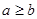and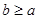, then a = b. Hence, ≥ is antisymmetric. Finally, ≥ is transitive sinceandimply that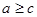. It follows that ≥ is a partial ordering on the set of integers andis a poset.

Example. The divisibility relation | is a partial ordering on the set of positive integers, since it is reflexive, antisymmetric and transitive. We see that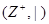is a poset (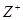denotes the set of positive integers).

Example. Show that the inclusion relation Í is a partial ordering on the power set of a set S.

Solution: Since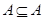whenever A is a subset of S, Í is reflexive. It is antisymmetric sinceand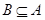imply that A = B. Finally, Í is transitive, sinceandimply that. Hence, Í is a partial ordering on P(S), andis a poset.

In a poset the notationdenotes that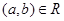. The notation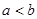denotes that, but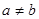. Also, we say “a is less than b” or “b is greater than a” if.

The elements a and b of a poset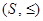are called comparable if eitheror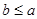. When a and b are elements of S such that neithernor, a and b are called incomparable.

Example. In the poset, are the integers 3 and 9 comparable? Are 5 and 7 comparable?

Solution: The integers 3 and 9 are comparable, since 3 | 9. The integers 5 and 7 are incomparable, because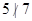and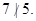The adjective “partial” is used to describe partial orderings since pairs of elements may be incomparable. When every two elements in the set are comparable, the relation is called a total ordering.

Ifis a poset and every two elements of S are comparable, S is called a totally ordered or linearly ordered set, and ≤ is called a total order or a linear order. A totally ordered set is also called a chain.

Example. The poset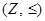is totally ordered, sinceorwhenever a and b are integers.

Example. The posetis not totally ordered since it contains elements that are incomparable, such as 5 and 7.is a well-ordered set if it is a poset such that ≤ is a total ordering and such that every nonempty subset of S has a least element.

Example. The set of ordered pairs of positive integers,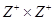, withif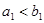, or if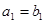and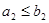(the lexicographic ordering), is a well-ordered set. The set Z, with the usual ≤ ordering, is not well-ordered since the set of negative integers, which is a subset of Z, has no least element.

Date: 2015-01-02; view: 781

 <== previous page | next page ==> Equivalence relations | Lexicographic order
doclecture.net - lectures - 2014-2020 year. Copyright infringement or personal data (0.002 sec.)